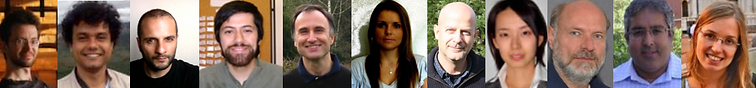top of page

# Título do Site

## universality from stochastic systems

Proposal summary

A classical problem in the field of interacting particle systems (IPS) is to derive the macroscopic laws of the thermodynamical quantities of a physical system by considering an underlying microscopic dynamics which is composed of particles that move according to some prescribed stochastic, or deterministic, law. The macroscopic laws can be partial differential equations (PDE) or stochastic PDE (SPDE) depending on whether one is looking at the convergence to the mean or to the fluctuations around that mean.

One of the purposes of this research project is to give a mathematically rigorous description of the derivation of SPDE from different IPS. We will focus on the derivation of the stochastic Burgers equation (SBE) and its integrated counterpart, namely, the KPZ equation, as well as their fractional versions. The KPZ equation is conjectured to be a universal SPDE describing the fluctuations of randomly growing interfaces of 1d stochastic dynamics close to a stationary state. With this study we want to characterize what is known as the KPZ universality class: the weak and strong conjectures. The latter states that there exists a universal process, namely the KPZ fixed point, which is a fixed point of the renormalization group operator of spacetime scaling 1:2:3, for which the KPZ is also invariant. The former states that the fluctuations of a large class of 1d conservative microscopic dynamics are ruled by stationary solutions of the KPZ.

Our goal is threefold: first, to derive the KPZ equation from general weakly asymmetric systems, showing its universality; second, to derive new SPDE, which are less studied in the literature, as the fractional KPZ from IPS which allow long jumps, the KPZ with boundary conditions from IPS in contact with reservoirs or with defects, and coupled KPZ from IPS with more than one conserved quantity. Finally, we will analyze the fluctuations of purely strong asymmetric systems, which are conjectured to be given by the KPZ fixed point.

## In case you are interested in applying for post-doctoral grants please clickbottom of page